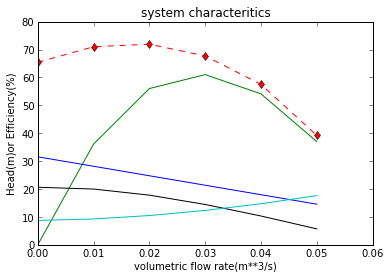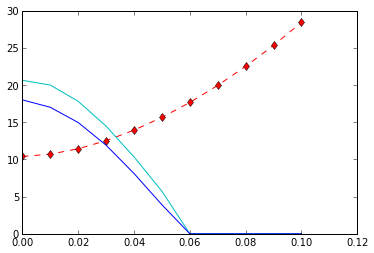# Chapter 6 : pumping of liquids¶

### example 6.1 page no : 115¶

In :
import math
from numpy import *

# Initialization of Variable
atp = 100.2*1000.
g = 9.81
rho_w = 996.
rho_toluene = 867.
vap_pre_toluene = 4.535*1000
viscosity_toluene = 5.26/10000

#calculation
m = (atp-vap_pre_toluene)/rho_toluene/g
print "Max. height of toluene supported by atm. pressure (in m): %.4f"%m

#part(1)
hopw = 0.650           #head of pump in terms of water
hopt = hopw*rho_w/rho_toluene           #head of pump in terms of toluene
Q = 1.8*10**-3                          #flow in m**3/s
d = 2.3*10**-2                          #diameter of pipe
pi = 3.14127

#u = 4*Q/pi/d**2
#substituting this for reynolds no.
Re = 4*Q*rho_toluene/pi/d/viscosity_toluene         #reynolds no.
print "reynolds no : %.4f"%Re
phi = 0.0396*Re**-0.25

#since both LHS and RHS are function of x(max. ht. ab. toluene)
#we define a new variable to solve the eqn
#y = (atp/rho_toluene/g)-(vap_pre_toluene/rho_toluene/g)-(4*phi*16*Q**2*x/pi**2/d**5/g)-hopt
#y = x
#these are two equations

b = array([0,((atp/rho_toluene/g)-(vap_pre_toluene/rho_toluene/g)-hopt)])
A = array([[1, -1],[1, 4*phi*16*Q**2/pi**2/d**5/g]])
x = linalg.solve(A ,b)
print "the maximum height above toulene in the tank the pump can be \
located without risk while flow rate is 1.80dm**3/s (in m): %.4f"%x

#solution of part(2)
l = 9.          #length
u = math.sqrt(((atp/rho_toluene/g)-(vap_pre_toluene/rho_toluene/g)-hopt-l)*d*g/4/phi/l)     #fluid velocity in pipes
Q = pi*d**2*u/4
print "Maximum delivery rate if pump is located 9m above toluene tank(in m**3/s) %.4f"%Q

#solution of part(3)
#clubing d together we get
Q = 1.8/1000.
a = (atp/rho_toluene/g)-(vap_pre_toluene/rho_toluene/g)-hopt-l
b = a*pi**2*g/4./9./16./Q**2/0.0396/(4*Q*rho_toluene/pi/viscosity_toluene)**-0.25
d = (1./b)**(1./4.75)
print "minimum smooth diameter of suction pipe which will have flow \
rate as (1.8 dm**3/s) for pump kept at 9 m high (in m):",d

Max. height of toluene supported by atm. pressure (in m): 11.2477
reynolds no : 164260.3512
the maximum height above toulene in the tank the pump can be located without risk while flow rate is 1.80dm**3/s (in m): 6.3463
Maximum delivery rate if pump is located 9m above toluene tank(in m**3/s) 0.0009
minimum smooth diameter of suction pipe which will have flow rate as (1.8 dm**3/s) for pump kept at 9 m high (in m): 0.0306728431855


### example 6.2 pageno : 118¶

In :
import math
# Initialization of Variable
Q1 = 24.8/1000          #flow in pump 1
d1 = 11.8/100           #diameter of impeller 1
H1 = 14.7               #head of pump 1
N1 = 1450.              #frequency of motor 1
Q2 = 48/1000.           #flow in pump 2

#calculation
H2 = 1.15*H1            #head of pump 2
specific_speed = N1*Q1**0.5/H1**0.75
N2 = specific_speed*H2**0.75/Q2**0.5        #frequency of motor 2
print "frequency of motor 2 in rpm %.4f"%N2
d2 = math.sqrt(N2**2*H1/H2/N1**2/d1**2)
print  "diametr of impeller 2 (in m) %.4f"%(1/d2 )

frequency of motor 2 in rpm 1157.4350
diametr of impeller 2 (in m) 0.1585


### example 6.3 page no : 120¶

In :
from matplotlib.pyplot import *
import math
%matplotlib inline

# Initialization of Variable
Q = [0, 0.01, 0.02, 0.03 ,0.04, 0.05]           #discharge
effi_hyd = [65.4 ,71, 71.9, 67.7, 57.5, 39.2]
effi_over = [0 ,36.1, 56.0, 61.0, 54.1, 37.0]
H_sys = [0 ,0, 0, 0, 0, 0]
d = 0.114           #diameter of pipe
d_o = 0.096         #diameter of impeller
h = 8.75            #elevation
g = 9.81            #acc. of gravity
rho = 999.          #denisity of water
l = 60.             #length of pipe
theta = 0.611       #angle in radians
B = 0.0125          #width of blades
pi = 3.1412
mu = 1.109/1000     #viscosity of water
omega = 2*pi*1750/60.
H_theor = []
# calculation
for i in range(6):
if i == 0:
H_sys[i] = h
else:
H_sys[i] = h+8.*Q[i]**2./pi**2/d**4/g*(1+8*l*0.0396/d*(4*rho*Q[i]/pi/d/mu)**-0.25)
H_theor.append(omega**2*d_o**2/g-omega*Q[i]/2/pi/g/B/math.tan(theta))

#H_theor = omega**2*d_o**2/g-omega*Q/2/pi/g/B/math.tan(theta)
#print (H_sys"head of system (in m)")
#print (H_theor)
H_eff = [0,0,0,0,0,0]
for i in range(6):
H_eff[i] = effi_hyd[i]*H_theor[i]/100.

#print (H_eff)
plot(Q,effi_hyd, 'r--d')
plot(Q,effi_over, 'g')
plot(Q,H_eff,'k')
plot(Q,H_theor)
plot(Q,H_sys ,'c-')
title('system characteritics')
xlabel('volumetric flow rate(m**3/s)')
show()

#calculation of power
#at intersecting point using datatrip b/w H_sys &H_eff
Q = 0.0336
effi_over = 59.9
H_eff = 13.10
P = H_eff*rho*g*Q/effi_over/10
print "Power required to pump fluid at this rate(in KW): %.4f"%P

Populating the interactive namespace from numpy and matplotlib

WARNING: pylab import has clobbered these variables: ['draw_if_interactive', 'pi', 'new_figure_manager']
%pylab --no-import-all prevents importing * from pylab and numpyPower required to pump fluid at this rate(in KW): 7.2014


### example 6.4 pageno : 123¶

In :
import math
from matplotlib.pyplot import *

# Initialization of Variable
#each is increased by five units to make each compatible for graph plotting
Q = [0, 0.01, 0.02, 0.03, 0.04, 0.05, 0.06, 0.07, 0.08, 0.09, 0.1]      #flow rate
HeffA = [20.63 ,19.99, 17.80, 14.46, 10.33, 5.71, 0, 0, 0, 0, 0 ]                 #Heff of pump A
HeffB = [18 ,17, 14.95, 11.90, 8.10, 3.90, 0, 0, 0, 0, 0]                         #Heff of pump B
alpha = 1.
h = 10.4
d = 0.14
l = 98.
pi = 3.1412
g = 9.81
rho = 999.
mu = 0.001109
H_sys = [0,0,0,0,0,0,0,0,0,0,0]
for i in range(11):
if i == 0:
H_sys[i] = h
else:
H_sys[i] = h+8*Q[i]**2/pi**2/d**4/g*(1+8*l*0.0396/d*(4*rho*Q[i]/pi/d/mu)**-0.25)

#H_sys is head of the system
print  "the head of system in terms of height of water : ",H_sys
plot(Q,H_sys,'r--d')
plot(Q,HeffA ,'-c')
plot(Q,HeffB)
show()

#at intersecting point using datatrip b/w H_sys &H_effA
print "the flow rate at which H_sys takes over HeffA ",0.03339

the head of system in terms of height of water :  [10.4, 10.703503558535079, 11.434551925145852, 12.522017190713953, 13.934570851357016, 15.652293146483034, 17.66081196342499, 19.949010875049055, 22.507899154909985, 25.329974605787854, 28.4088306047139]the flow rate at which H_sys takes over HeffA  0.03339


### example 6.5 pageno : 126¶

In :
import math
from numpy import *

# Initialization of Variable
rho = 1000.
dc = .15
l = 7.8
g = 9.81
pi = 3.1428
atp = 105.4*1000
vap_pre = 10.85*1000
sl = .22
dp = 0.045
h = 4.6

#("x(t) = sl/2*cos(2*pi*N*t)"  "the function of print lcement")
#"since we have to maximize the acceleration double derivate the terms")
#since double derivation have the term cos(kt)
#finding it maxima
t = linspace(0,5,100)
k = 1.

def  maximacheckerforcosine():
h = 0.00001
a = 0.00
for i in range(1,401):
if (math.cos(a+h)-math.cos(a-h))/2*h == 0 and math.cos(i-1)>0:
break
else:
a = 0.01+a
m = i-1
v = math.cos(i-1)
return m,v

a, b =  maximacheckerforcosine()

print "time t when the acceleration will be maximum(s)",a

#double derivative will result in a square of value of N
#lets consider its coefficient all will be devoid of N**2
k = sl/2*(2*pi)**2                      #accn max of piston
kp = k*1./4*pi*dc**2/1.*4/pi/dp**2      #accn coeff. ofsuction pipe
f = 1./4*pi*dp**2*l*rho*kp              #force exerted by piston
p = f/1.*4./pi/dp**2                    #pressure exerted by piston

#calculation
o = atp-h*rho*g-vap_pre
y = poly1d([-p, 0,o],False)
a = roots(y)
print "Maximum frequency of oscillation if cavitation o be avoided(in Hz) %.4f"%abs(a)

time t when the acceleration will be maximum(s) 0
Maximum frequency of oscillation if cavitation o be avoided(in Hz) 0.3622


### example 6.6 pageno : 128¶

In :
import math

# Initialization of Variable
rhos = 1830.            #density of acid
atp = 104.2*1000        #atmospheric pressure
temp = 11.+273          #temp in kelvin
M = 28.8/1000           #molar mass of air
R = 8.314               #universal gas constant
g = 9.81                #acceleration of gravity
pi = 3.14
d = 2.45                #diameter of tank
l = 10.5                #length of tank
h_s = 1.65              #height of surface of acid from below
effi = 0.93             #efficiency

#calculation
mliq = pi*d**2*l*rhos/4
h_atm = atp/rhos/g      #height conversion of atp
h_r = 4.3-1.65          #height difference
mair = g*h_r*mliq*M/(effi*R*temp*math.log(h_atm/(h_atm+h_s)))       #mass of air
print "mass of air required to lift the sulphuric acid tank %.4f"%mair
print "The negative sign indicates air is expanding & work done is magnitude of value in kg:"
m = abs(mair/mliq)
print  "The mass of air required for per kilo of acid transferred: %.4f"%m

mass of air required to lift the sulphuric acid tank -123.3851
The negative sign indicates air is expanding & work done is magnitude of value in kg:
The mass of air required for per kilo of acid transferred: 0.0014

In [ ]: